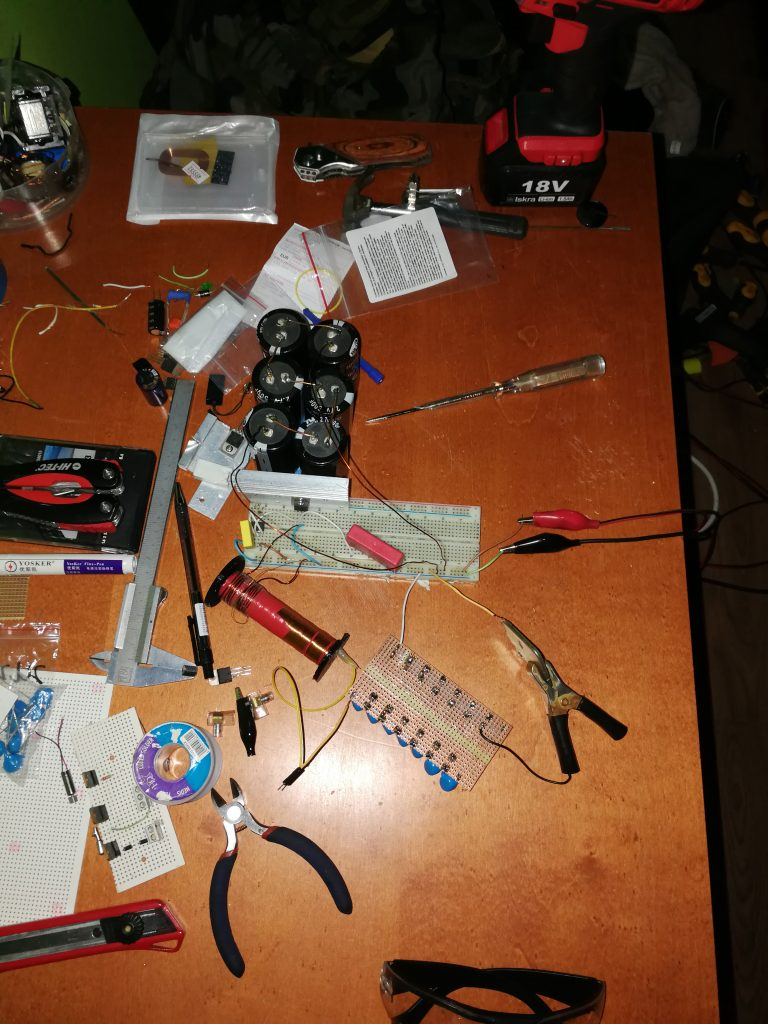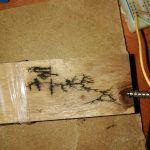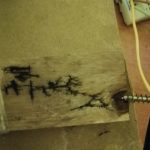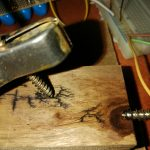# High voltage circuit

First I made the frequency generator with the ne555n timer, then I put the transistor IRF840 to work and made the transformer for the output.Than I put the HV (640V) capacitor on the leads of the transformer primary winding and that was it for the first step.

Then I made a second circuit with the capacitors and diodes for voltage multiplication.

The voltage multiplication is 11x so the output voltage should be around (1000 x 11) 11 000 V or 11kV

Lichtenberg figures with the HV circuit driver.Lichtenberg figuresLichtenberg figuresLichtenberg figuresLichtenberg figures dark